JMSLTM Numerical Library 7.2.0
com.imsl.math

Class ODE

• Field Summary

Fields
Modifier and Type Field and Description
static int AFTER_SUCCESSFUL_STEP
Used by method examineStep to indicate examining after a successful step
static int AFTER_UNSUCCESSFUL_STEP
Used by method examineStep to indicate examining after an unsuccessful step
static int BEFORE_STEP
Used by method examineStep to indicate examining before the next step
static int ERROR_NORM_ABS
Used by method setNorm to indicate that the error norm to be used is to be the absolute error, equals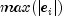static int ERROR_NORM_EUCLIDEAN
Used by method setNorm to indicate that the error norm to be used is to be the scaled Euclidean norm defined as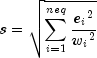static int ERROR_NORM_MAX
Used by method setNorm to indicate that the error norm to be used is to be the maximum of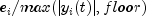where floor is set via setFloor
static int ERROR_NORM_MINABSREL
Used by method setNorm to indicate that the error norm to be used is to be the minimum of the absolute error and the relative error, equals the maximum of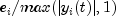• Constructor Summary

Constructors
Constructor and Description
ODE()
• Method Summary

Methods
Modifier and Type Method and Description
protected void examineStep(int state, double t, double[] y)
Called before and after each internal step.
double getFloor()
Returns the value used in the norm computation.
double getInitialStepsize()
Returns the initial internal step size.
double getMaximumStepsize()
Returns the maximum internal step size.
int getMaxSteps()
Returns the maximum number of internal steps allowed.
double getMinimumStepsize()
Returns the minimum internal step size.
int getNorm()
Returns the switch for determining the error norm.
double getScale()
Returns the scaling factor.
double getTolerance()
Returns the error tolerance.
void setFloor(double floor)
Sets the value used in the norm computation.
void setInitialStepsize(double stepsize)
Sets the initial internal step size.
void setMaximumStepsize(double stepsize)
Sets the maximum internal step size.
void setMaxSteps(int maxSteps)
Sets the maximum number of internal steps allowed.
void setMinimumStepsize(double stepsize)
Sets the minimum internal step size.
void setNorm(int normMethod)
Sets the switch for determining the error norm.
void setScale(double scale)
Sets the scaling factor.
void setTolerance(double tolerance)
Sets the error tolerance.
protected double vnorm(double[] v, double[] y, double[] ymax)
Returns the norm of a vector.
• Field Detail

• AFTER_SUCCESSFUL_STEP

public static final int AFTER_SUCCESSFUL_STEP
Used by method examineStep to indicate examining after a successful step
Constant Field Values
• AFTER_UNSUCCESSFUL_STEP

public static final int AFTER_UNSUCCESSFUL_STEP
Used by method examineStep to indicate examining after an unsuccessful step
Constant Field Values
• BEFORE_STEP

public static final int BEFORE_STEP
Used by method examineStep to indicate examining before the next step
Constant Field Values
• ERROR_NORM_ABS

public static final int ERROR_NORM_ABS
Used by method setNorm to indicate that the error norm to be used is to be the absolute error, equalsConstant Field Values
• ERROR_NORM_EUCLIDEAN

public static final int ERROR_NORM_EUCLIDEAN
Used by method setNorm to indicate that the error norm to be used is to be the scaled Euclidean norm defined aswhere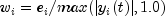and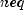is the number of equations
Constant Field Values
• ERROR_NORM_MAX

public static final int ERROR_NORM_MAX
Used by method setNorm to indicate that the error norm to be used is to be the maximum ofwhere floor is set via setFloor
Constant Field Values
• ERROR_NORM_MINABSREL

public static final int ERROR_NORM_MINABSREL
Used by method setNorm to indicate that the error norm to be used is to be the minimum of the absolute error and the relative error, equals the maximum ofConstant Field Values
• Constructor Detail

• ODE

public ODE()
• Method Detail

• examineStep

protected void examineStep(int state,
double t,
double[] y)
Called before and after each internal step. This method can be over-ridden by the user to examine intermediate values of t and y.
Parameters:
state - an int, one of BEFORE_STEP, AFTER_SUCCESSFUL_STEP or AFTER_UNSUCCESSFUL_STEP.
t - double representing the independent variable.
y - double array containing the dependent variables.
• getFloor

public double getFloor()
Returns the value used in the norm computation.
Returns:
a double used in the norm computation.
• getInitialStepsize

public double getInitialStepsize()
Returns the initial internal step size.
Returns:
a double specifying the initial internal step size.
• getMaximumStepsize

public double getMaximumStepsize()
Returns the maximum internal step size.
Returns:
a double specifying the maximum internal step size.
• getMaxSteps

public int getMaxSteps()
Returns the maximum number of internal steps allowed.
Returns:
an int specifying the maximum number of internal steps allowed.
• getMinimumStepsize

public double getMinimumStepsize()
Returns the minimum internal step size.
Returns:
a double specifying the minimum internal step size.
• getNorm

public int getNorm()
Returns the switch for determining the error norm.
Returns:
an int specifying the switch for determining the error norm. In the following,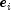is the absolute value for an estimate of the error in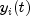.

 normMethod Constraint ERROR_NORM_MINABSREL Minimum of the absolute error and the relative error, equals the maximum ofERROR_NORM_ABS Absolute error, equals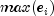ERROR_NORM_MAX Maximum ofERROR_NORM_EUCLIDEAN Scaled Euclidean norm defined aswhereandis the number of equations

• getScale

public double getScale()
Returns the scaling factor.
Returns:
a double specifying the scaling factor.
• getTolerance

public double getTolerance()
Returns the error tolerance.
Returns:
a double specifying the error tolerance.
• setFloor

public void setFloor(double floor)
Sets the value used in the norm computation.
Parameters:
floor - a double used in the norm computation. floor must be greater than zero.
Default: floor = 1.0
• setInitialStepsize

public void setInitialStepsize(double stepsize)
Sets the initial internal step size.
Parameters:
stepsize - a double specifying the initial internal step size. stepsize must be greater than or equal to zero. Default: stepsize = 0.0
• setMaximumStepsize

public void setMaximumStepsize(double stepsize)
Sets the maximum internal step size.
Parameters:
stepsize - a double specifying the maximum internal step size. stepsize must be greater than zero.
Default: See the setMaximumStepsize method in the subclasses for the default values used.
• setMaxSteps

public void setMaxSteps(int maxSteps)
Sets the maximum number of internal steps allowed.
Parameters:
maxSteps - an int specifying the maximum number of internal steps allowed. maxSteps must be greater than zero. Default: maxSteps = 500
• setMinimumStepsize

public void setMinimumStepsize(double stepsize)
Sets the minimum internal step size.
Parameters:
stepsize - a double specifying the minimum internal step size. stepsize must be greater than or equal to zero. Default: stepsize = 0.0
• setNorm

public void setNorm(int normMethod)
Sets the switch for determining the error norm.
Parameters:
normMethod - an int specifying the switch for determining the error norm.
Default: normMethod = ERROR_NORM_MINABSREL
In the following table,is the absolute value for an estimate of the error in.

 normMethod Constraint ERROR_NORM_MINABSREL Minimum of the absolute error and the relative error, equals the maximum ofERROR_NORM_ABS Absolute error, equalsERROR_NORM_MAX Maximum ofERROR_NORM_EUCLIDEAN Scaled Euclidean norm defined aswhereandis the number of equations

• setScale

public void setScale(double scale)
Sets the scaling factor.
Parameters:
scale - a double specifying the scaling factor. scale must be greater than zero.
Default: scale = 1.0
• setTolerance

public void setTolerance(double tolerance)
Sets the error tolerance.
Parameters:
tolerance - a double specifying the error tolerance. tolerance must be greater than zero. Default: tolerance = 1.0e-6
• vnorm

protected double vnorm(double[] v,
double[] y,
double[] ymax)
Returns the norm of a vector. This method can be over-ridden by the user to supply a different norm than those available through setNorm.
Parameters:
v - a double array containing the vector whose norm is to be computed
y - a double array containing the values of the dependent variable
ymax - a double array containing the maximum y values computed thus far
Returns:
a double scalar value representing the norm of the vector v
JMSLTM Numerical Library 7.2.0